# Electricity and Magnetism Electric Charges and Forces Electric

• Slides: 44Electricity and Magnetism Electric Charges and Forces Electric Charge Coulomb’s Law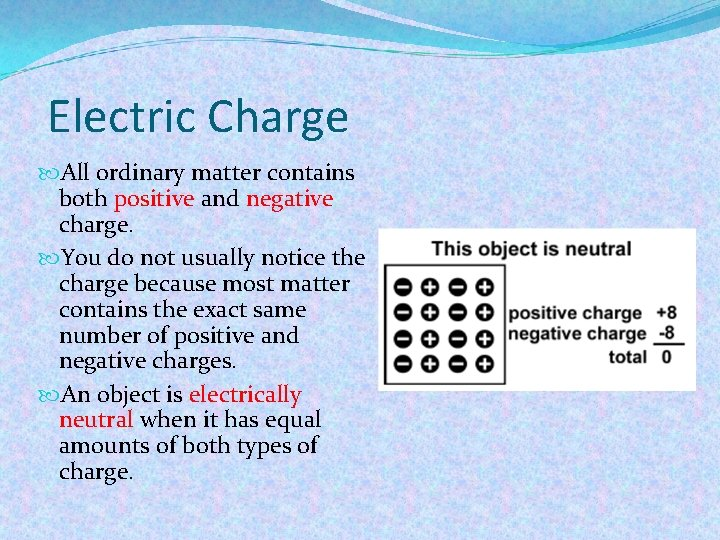Electric Charge All ordinary matter contains both positive and negative charge. You do not usually notice the charge because most matter contains the exact same number of positive and negative charges. An object is electrically neutral when it has equal amounts of both types of charge.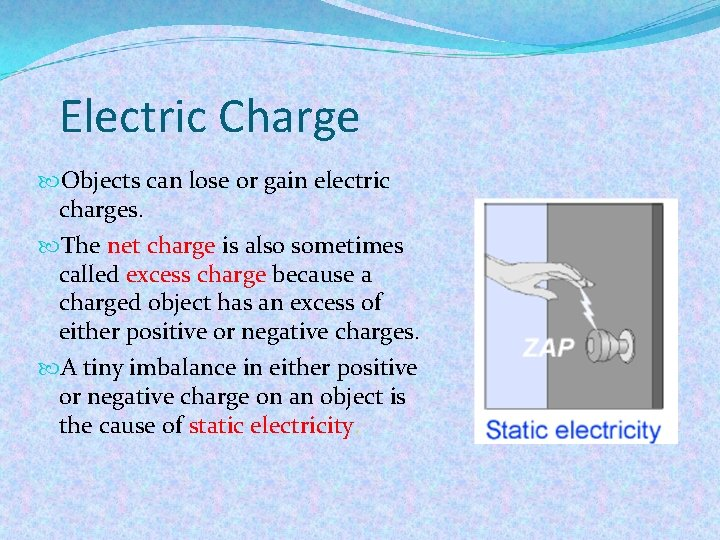Electric Charge Objects can lose or gain electric charges. The net charge is also sometimes called excess charge because a charged object has an excess of either positive or negative charges. A tiny imbalance in either positive or negative charge on an object is the cause of static electricity.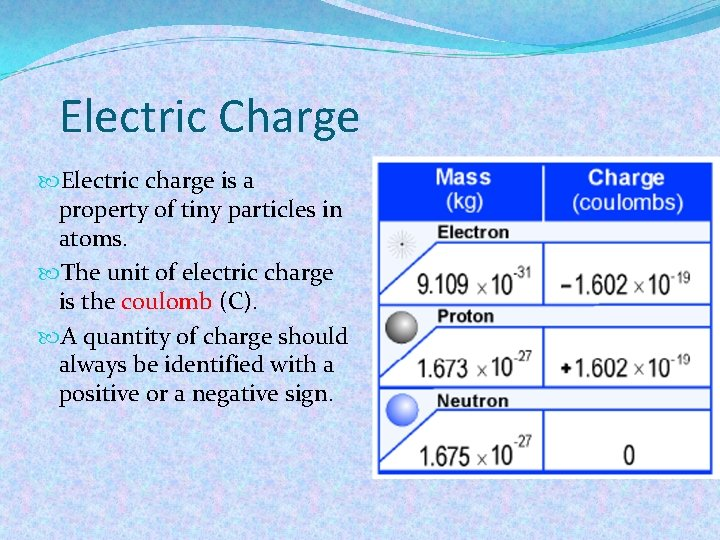Electric Charge Electric charge is a property of tiny particles in atoms. The unit of electric charge is the coulomb (C). A quantity of charge should always be identified with a positive or a negative sign.Electric forces are created between all electric charges. Because there are two kinds of charge (positive and negative) the electrical force between charges can attract or repel.Electric forces The forces between the two kinds of charge can be observed with an electroscope.Electric forces Charge can be transferred by conduction.Electric current § The direction of current was historically defined as the direction that positive charges move. § Both positive and negative charges can carry current. In conductive liquids (salt water) both positive and negative charges carry current. In solid metal conductors, only the electrons can move, so current is carried by the flow of negative electrons.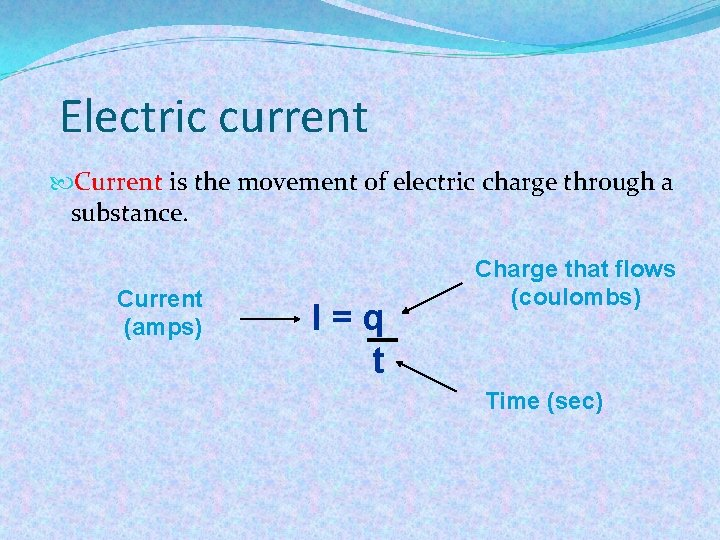Electric current Current is the movement of electric charge through a substance. Current (amps) I=q t Charge that flows (coulombs) Time (sec)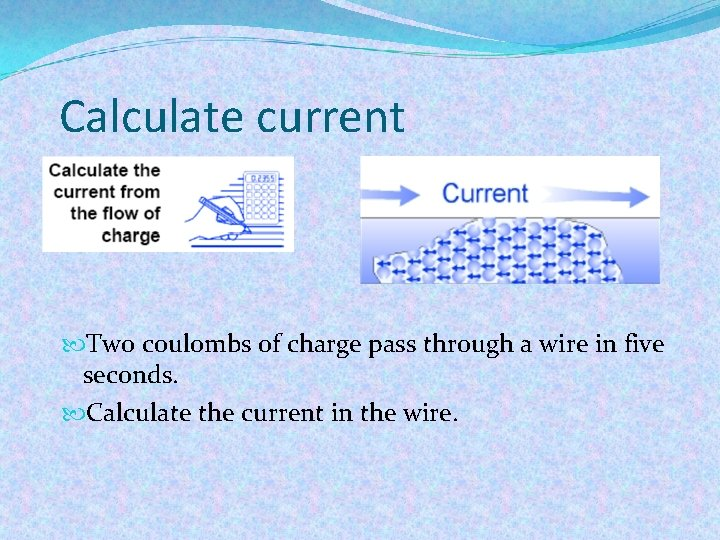Calculate current Two coulombs of charge pass through a wire in five seconds. Calculate the current in the wire.Conductors and insulators All materials contain electrons. The electrons are what carry the current in a conductor. The electrons in insulators are not free to move—they are tightly bound inside atoms.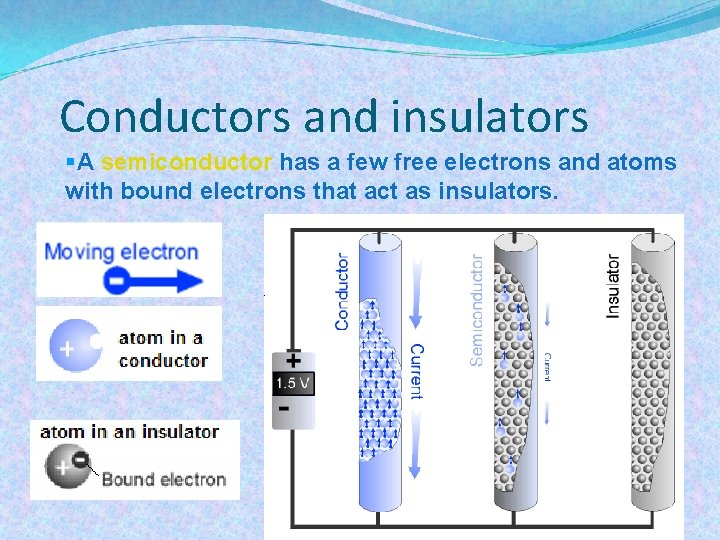Conductors and insulators §A semiconductor has a few free electrons and atoms with bound electrons that act as insulators.Conductors and insulators When two neutral objects are rubbed together, charge is transferred from one to the other and the objects become oppositely charged. This is called charging by friction. Objects charged by this method will attract each other.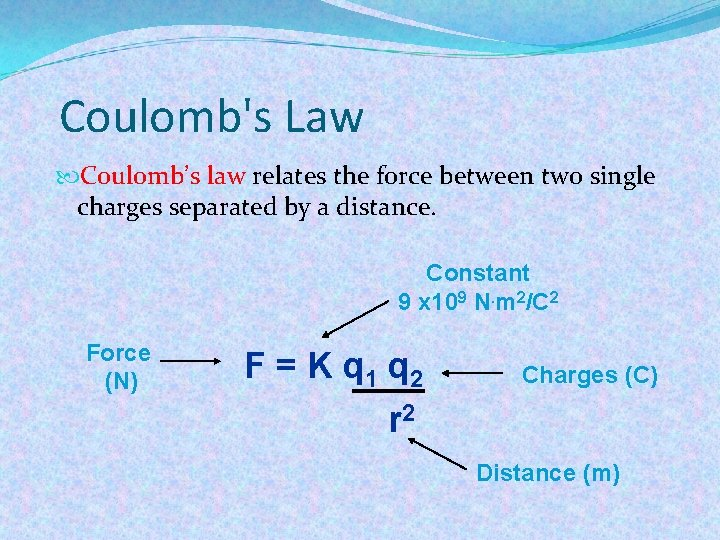Coulomb's Law Coulomb’s law relates the force between two single charges separated by a distance. Constant 9 x 109 N. m 2/C 2 Force (N) F = K q 1 q 2 Charges (C) r 2 Distance (m)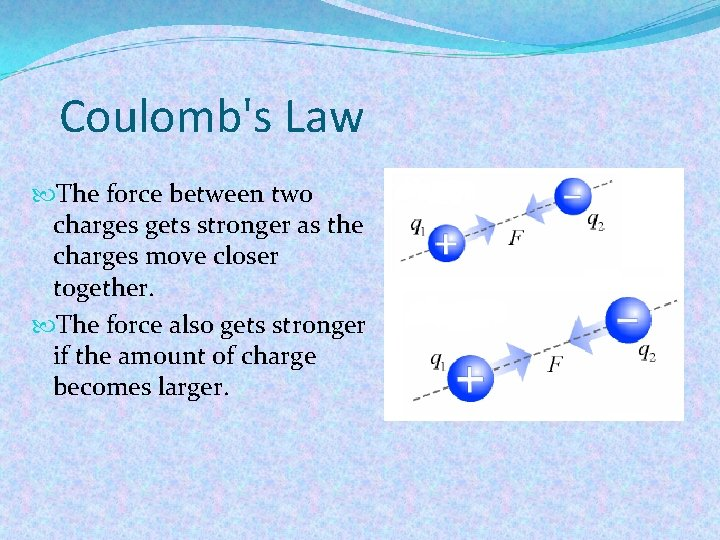Coulomb's Law The force between two charges gets stronger as the charges move closer together. The force also gets stronger if the amount of charge becomes larger.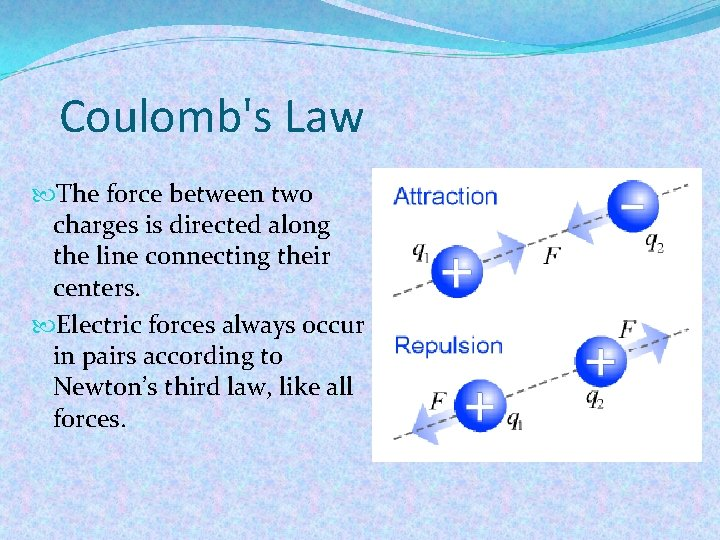Coulomb's Law The force between two charges is directed along the line connecting their centers. Electric forces always occur in pairs according to Newton’s third law, like all forces.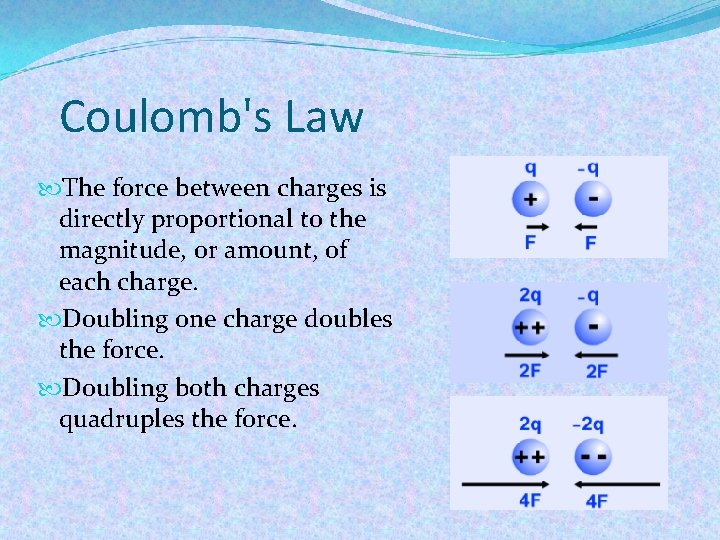Coulomb's Law The force between charges is directly proportional to the magnitude, or amount, of each charge. Doubling one charge doubles the force. Doubling both charges quadruples the force.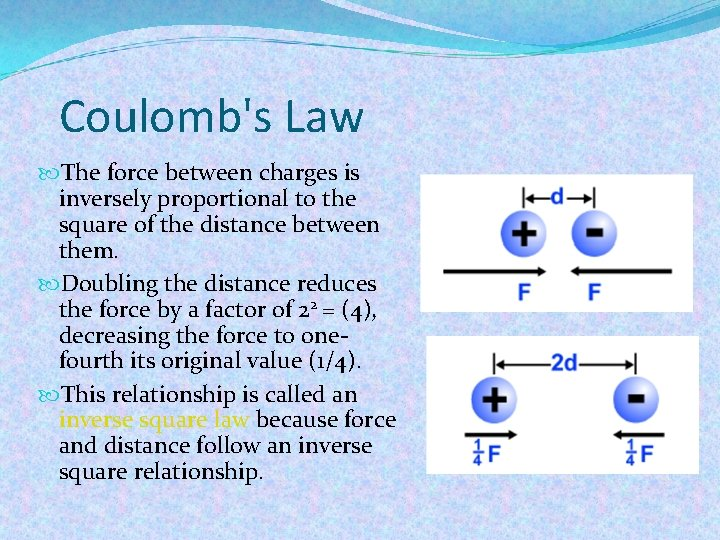Coulomb's Law The force between charges is inversely proportional to the square of the distance between them. Doubling the distance reduces the force by a factor of 22 = (4), decreasing the force to onefourth its original value (1/4). This relationship is called an inverse square law because force and distance follow an inverse square relationship.Calculating force Two balls are each given a static electric charge of one ten-thousandth (0. 0001) of a coulomb. Calculate the force between the charges when they are separated by one-tenth (0. 1) of a meter. Compare the force with the weight of an average 70 kg person.1) You are asked to calculate the force and compare it to a person’s weight. 2) You are given the charges and separation, and the mass of the person. 3) Use Coulomb’s law, F= -Kq 1 q 2/d 2, for the electric force and F=mg for the weight. 4) Solve: F = (9× 109 N • m 2/C 2)(0. 0001 C)(. 0001 C) ÷ (0. 1 m)2 = 9, 000 N The weight of a 70 kg person: F = mg = (70 kg)(9. 8 N/kg) = 686 N The force between the charges is 13. 1 times the weight of an average person (9, 000 ÷ 686).Fields and forces The concept of a field is used to describe any quantity that has a value for all points in space. You can think of the field as the way forces are transmitted between objects. Charge creates an electric field that creates forces on other charges.Fields and forces Mass creates a gravitational field that exerts forces on other masses.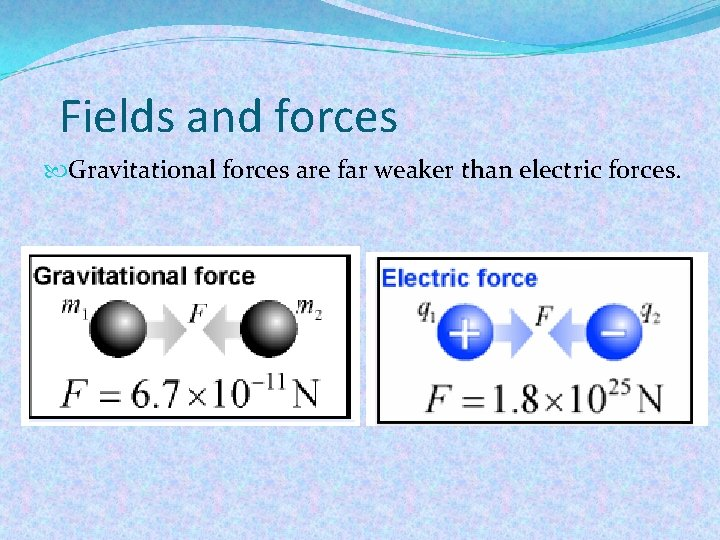Fields and forces Gravitational forces are far weaker than electric forces.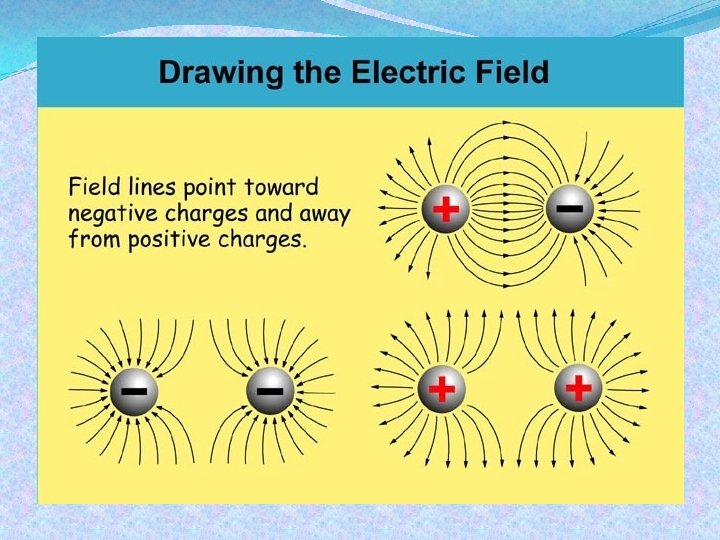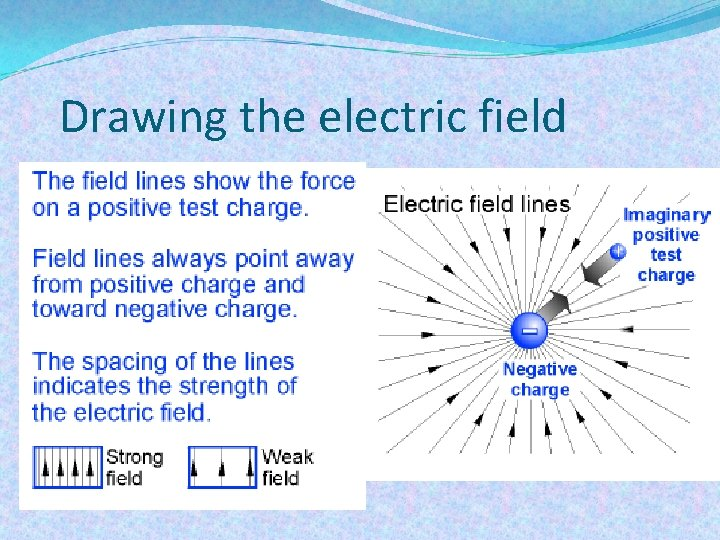Drawing the electric fieldElectric fields and electric force On the Earth’s surface, the gravitational field creates 9. 8 N of force on each kilogram of mass. With gravity, the strength of the field is in newtons per kilogram (N/kg) because the field describes the amount of force per kilogram of mass.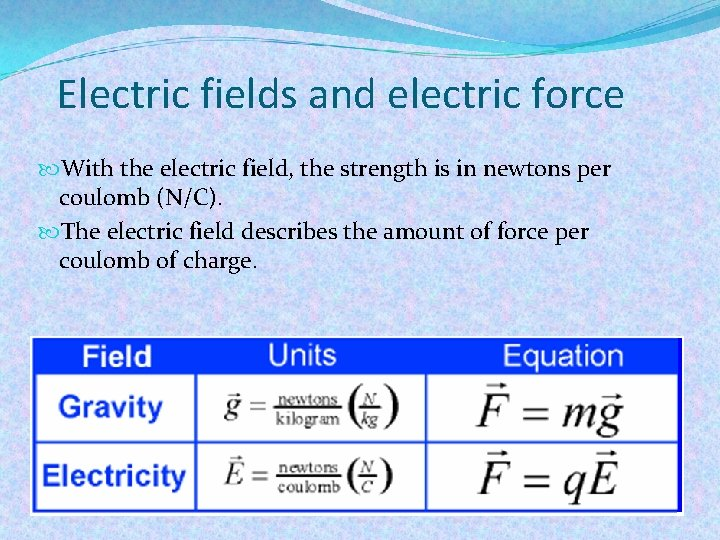Electric fields and electric force With the electric field, the strength is in newtons per coulomb (N/C). The electric field describes the amount of force per coulomb of charge.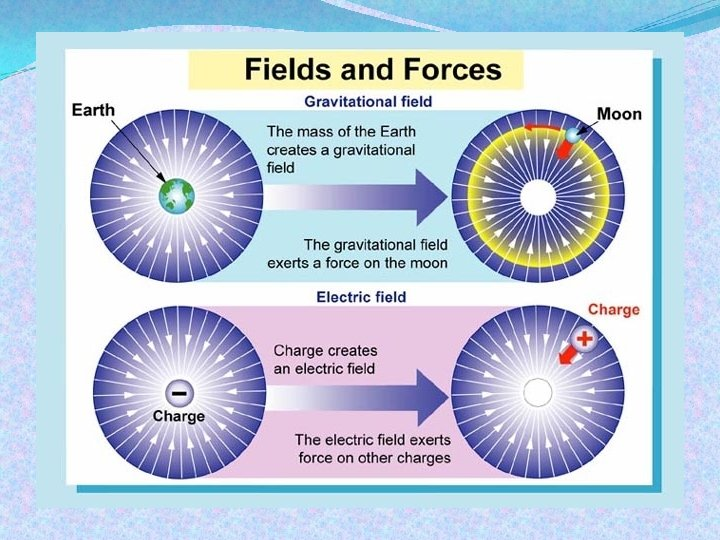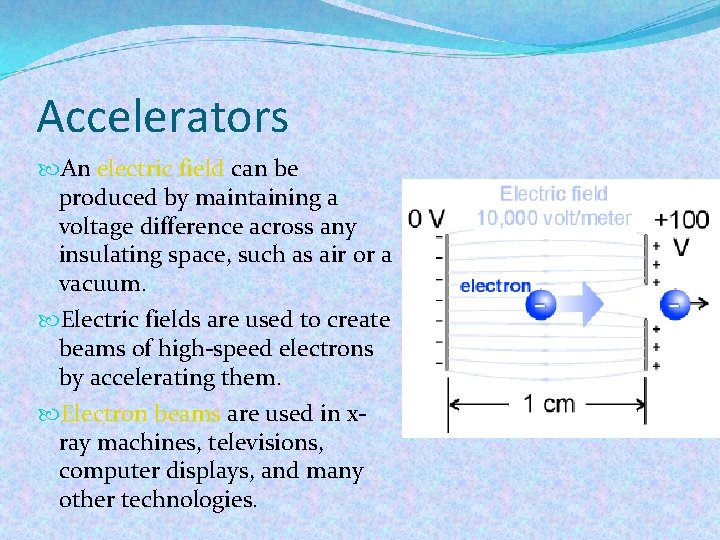Accelerators An electric field can be produced by maintaining a voltage difference across any insulating space, such as air or a vacuum. Electric fields are used to create beams of high-speed electrons by accelerating them. Electron beams are used in xray machines, televisions, computer displays, and many other technologies.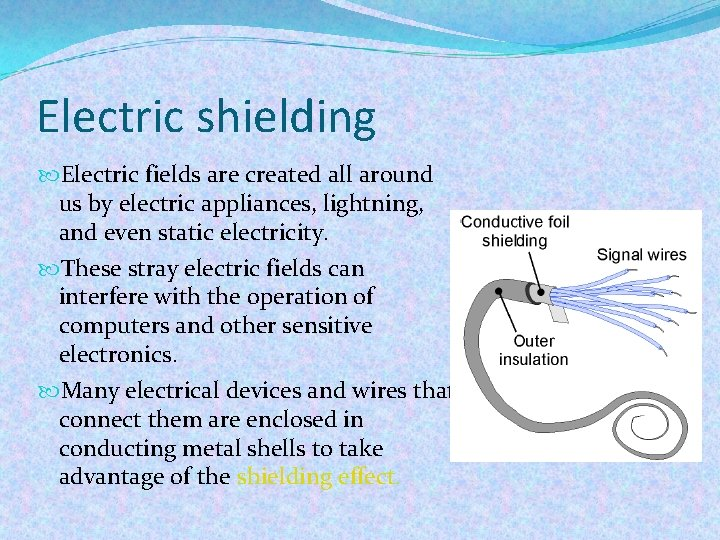Electric shielding Electric fields are created all around us by electric appliances, lightning, and even static electricity. These stray electric fields can interfere with the operation of computers and other sensitive electronics. Many electrical devices and wires that connect them are enclosed in conducting metal shells to take advantage of the shielding effect.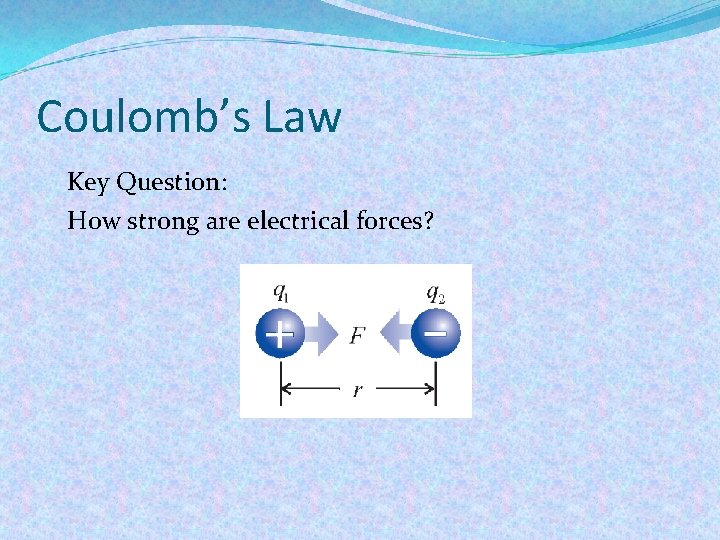Coulomb’s Law Key Question: How strong are electrical forces?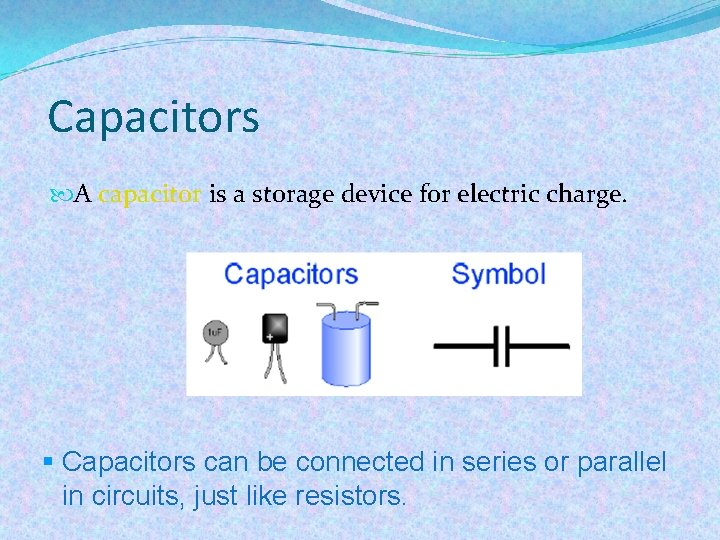Capacitors A capacitor is a storage device for electric charge. § Capacitors can be connected in series or parallel in circuits, just like resistors.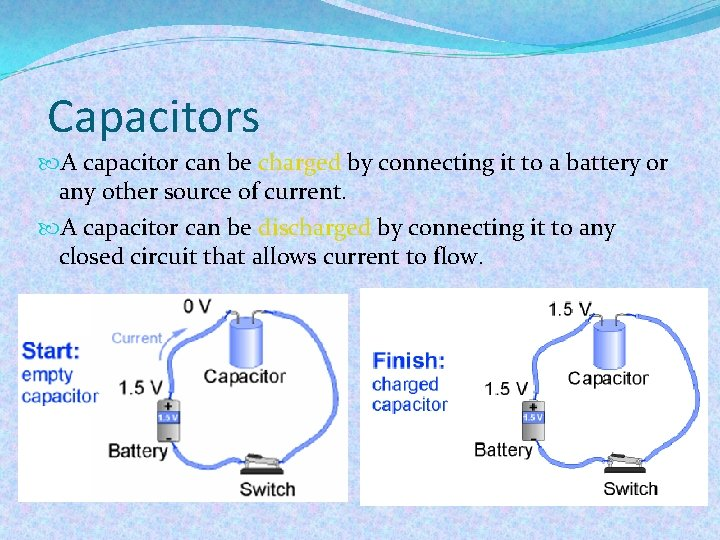Capacitors A capacitor can be charged by connecting it to a battery or any other source of current. A capacitor can be discharged by connecting it to any closed circuit that allows current to flow.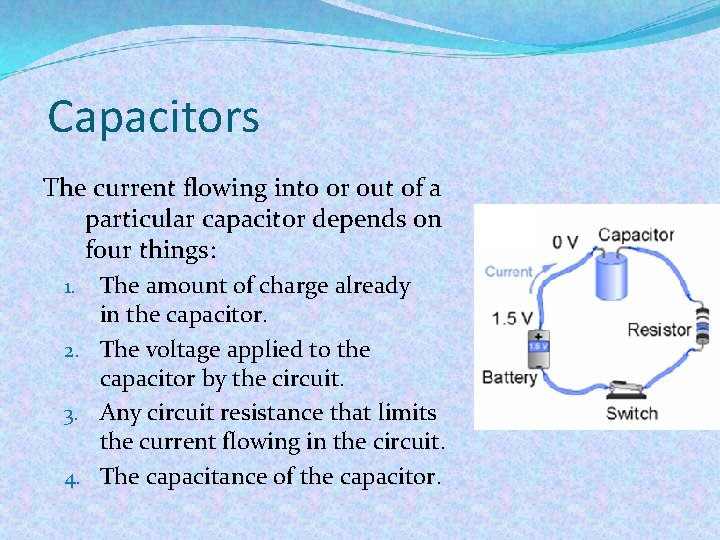Capacitors The current flowing into or out of a particular capacitor depends on four things: The amount of charge already in the capacitor. 2. The voltage applied to the capacitor by the circuit. 3. Any circuit resistance that limits the current flowing in the circuit. 4. The capacitance of the capacitor. 1.How a capacitor works inside The simplest type of capacitor is called a parallel plate capacitor. It is made of two conductive metal plates that are close together, with an insulating plate in between to keep the charges from coming together. Wires conduct charges coming in and out of the capacitor.How a capacitor works inside The amount of charge a capacitor can store depends on several factors: 1. The voltage applied to the capacitor. 2. The insulating ability of the material between the positive and negative plates. 3. The area of the two plates (larger areas can hold more charge). 4. The separation distance between the plates.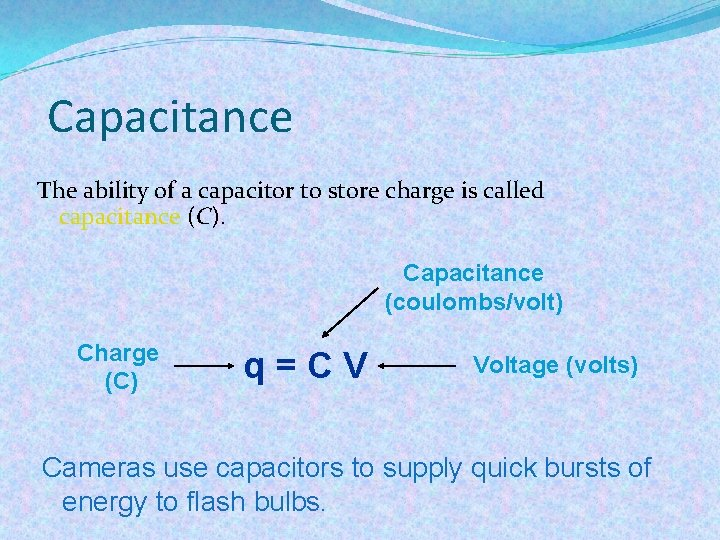Capacitance The ability of a capacitor to store charge is called capacitance (C). Capacitance (coulombs/volt) Charge (C) q=CV Voltage (volts) Cameras use capacitors to supply quick bursts of energy to flash bulbs.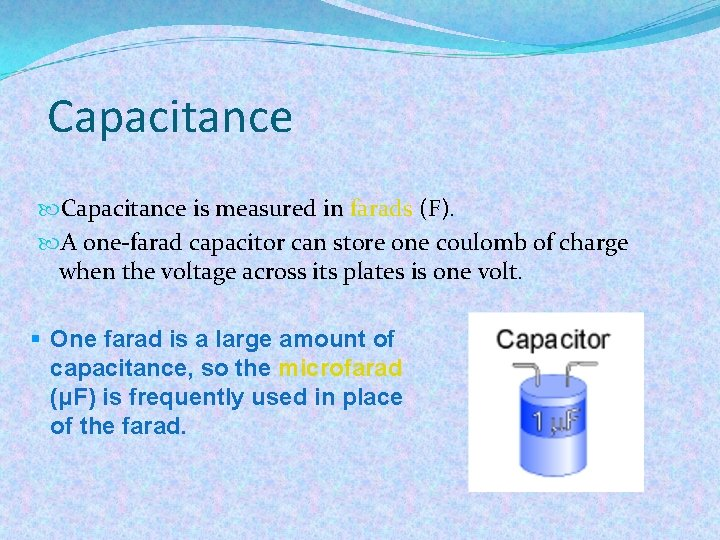Capacitance is measured in farads (F). A one-farad capacitor can store one coulomb of charge when the voltage across its plates is one volt. § One farad is a large amount of capacitance, so the microfarad (μF) is frequently used in place of the farad.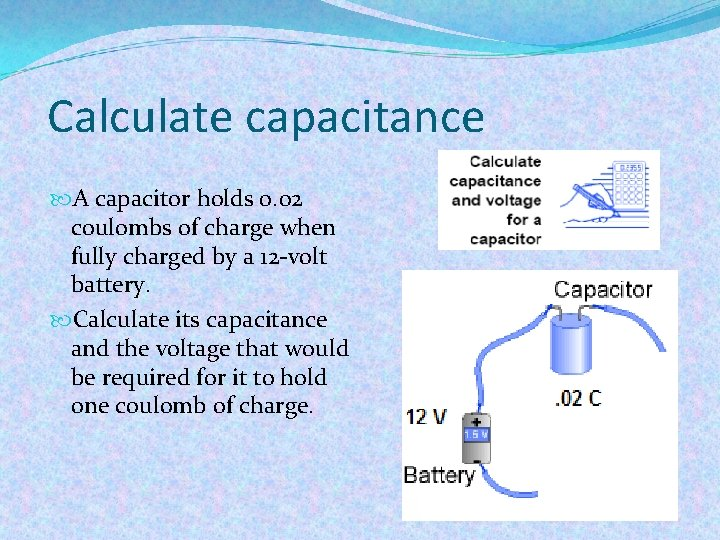Calculate capacitance A capacitor holds 0. 02 coulombs of charge when fully charged by a 12 -volt battery. Calculate its capacitance and the voltage that would be required for it to hold one coulomb of charge.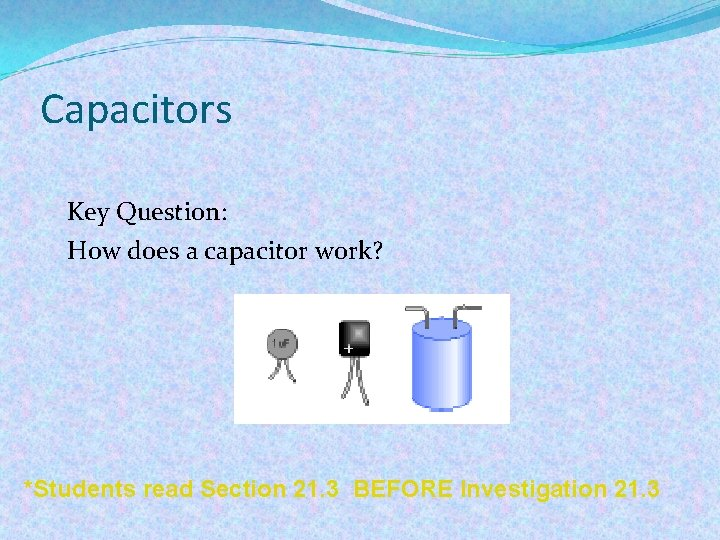Capacitors Key Question: How does a capacitor work? *Students read Section 21. 3 BEFORE Investigation 21. 3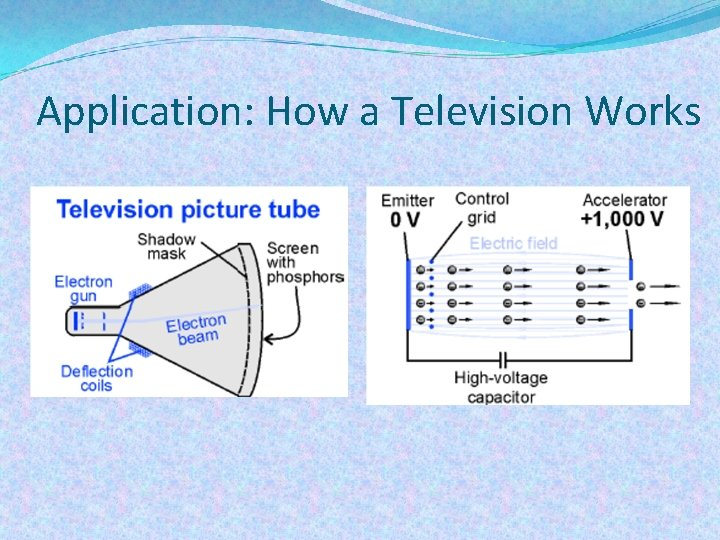Application: How a Television Works